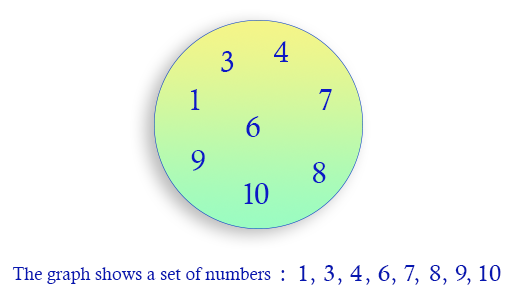﻿ Python: Create a new empty set - w3resource# Python: Create a new empty set

## Python sets: Exercise-1 with Solution

Write a Python program to create a set.

From Wikipedia,
In mathematics, a set is a collection of distinct elements. The elements that make up a set can be any kind of things: people, letters of the alphabet, numbers, points in space, lines, other geometrical shapes, variables, or even other sets. Two sets are equal if and only if they have precisely the same elementsTo create an empty set you have to use set(), not {}; the latter creates an empty dictionary, a data structure that we discuss in the next section.

Sample Solution:

Python Code:

``````print("Create a new set:")
x = set()
print(x)
print(type(x))
print("\nCreate a non empty set:")
n = set([0, 1, 2, 3, 4])
print(n)
print(type(n))
print("\nUsing a literal:")
a = {1,2,3,'foo','bar'}
print(type(a))
print(a)
```
```

Sample Output:

```Create a new set:
set()
<class 'set'>

Create a non empty set:
{0, 1, 2, 3, 4}
<class 'set'>

Using a literal:
<class 'set'>
{1, 2, 3, 'bar', 'foo'}
```

## Visualize Python code execution:

The following tool visualize what the computer is doing step-by-step as it executes the said program:

Python Code Editor:

Have another way to solve this solution? Contribute your code (and comments) through Disqus.

What is the difficulty level of this exercise?

Test your Python skills with w3resource's quiz

﻿

## Python: Tips of the Day

For-else construct useful when searched for something and find it:

```# For example assume that I need to search through a list and process each item until a flag item is found and
# then stop processing. If the flag item is missing then an exception needs to be raised.

for i in mylist:
if i == theflag:
break
process(i)
else:
raise ValueError("List argument missing terminal flag.")
```